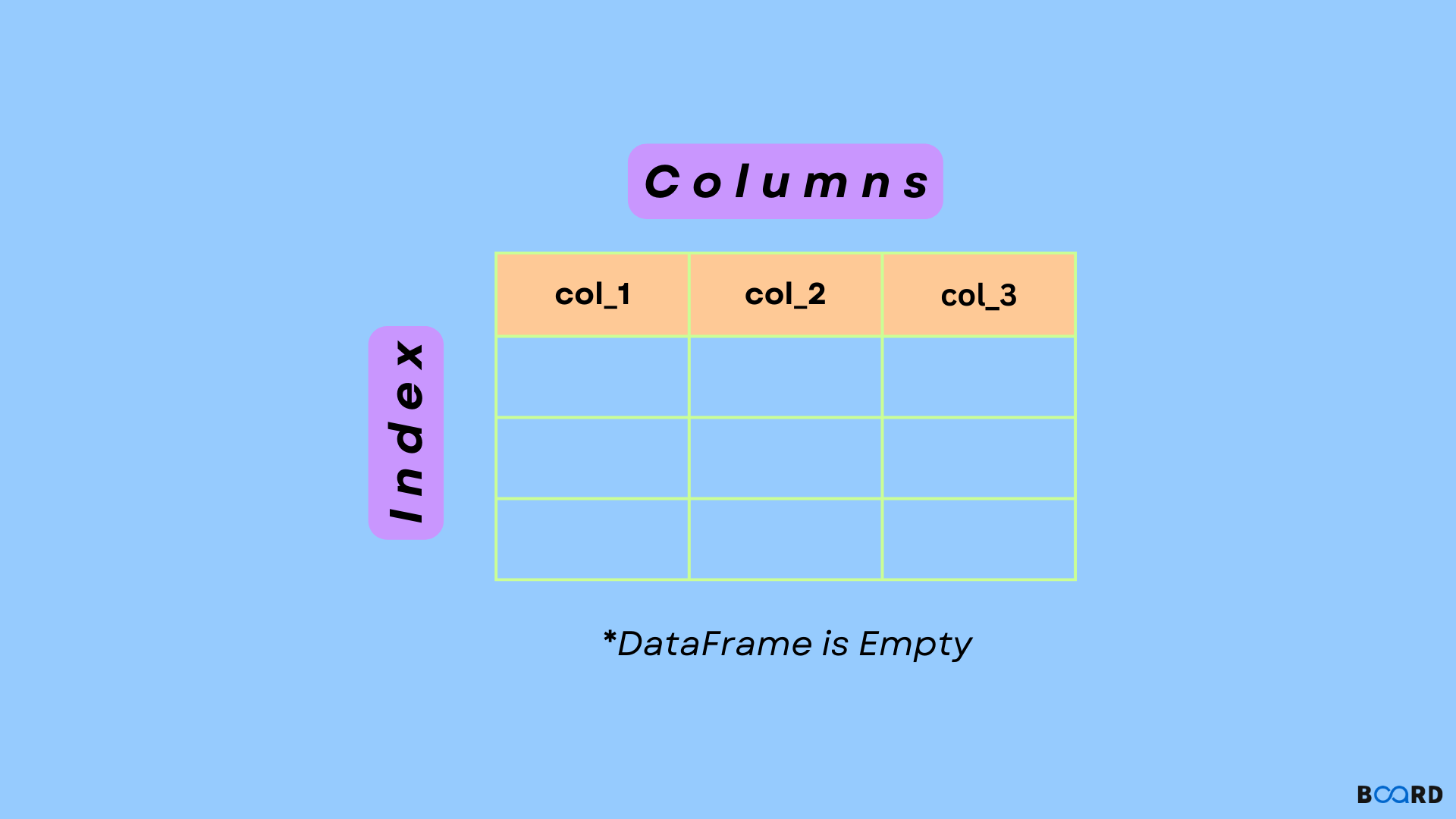# How to create an Empty Dataframe in Python using Pandas?## Introduction

Data Analysis involves a lot of Data Preprocessing before getting some insights from it. So, it becomes crucial to manipulate and prepare the datasets efficiently. Python, being so popular in Data Science offers Pandas, an open-source library for high-performance data manipulation.

Pandas has its own Data Structures- Series and DataFrames which are simple, fast, and efficient for Data Analysis. In this article, we will see how we can create an Empty DataFrame and use it to effectively load and manipulate our data.

A DataFrame is a Two-dimensional Data Structure with labeled axes called ‘Index’ and ‘Columns.’ This means that in DataFrame, datasets are arranged in the form of rows and columns. It can be seen as a dictionary of Series, which is a One-dimensional Array in Pandas used for storing various data types.

An important feature of DataFrame is that it is size-mutable, which means once you create an empty DataFrame, you can assign multiple columns to it later. Pandas DataFrame can also be created using other data structures of python such as List, Dictionary, etc.

For working with DataFrames, you need to install Pandas Library using the following command:

pip install pandas

Let’s look at the different methods for creating an empty DataFrame in Python.

## Using DataFrame Constructor

It is the most fundamental way of creating a DataFrame in Python using the following code.

 # Import the pandas libraryimport pandas as pd# Call Dataframe Constructordf1 = pd.DataFrame()# Print the Dataframe Objectprint(df1)

Let’s see how this code works!

 Empty DataFrameColumns: []Index: []

Firstly, Pandas library is imported with the alias of ‘pd.’ Then, we call the DataFrame Constructor to create an empty DataFrame Object. This DataFrame Object is assigned to variable ‘df’ which is printed using the print statement.

write your code here: Coding Playground

## Using a Python List

This method is used when you have to create a DataFrame using a python list. While implementing this method, you have to pass the list as an argument to the DataFrame function.

 data =[]df2 = pd.DataFrame(data, index=[], columns=[])print(df2)

Output:

 Empty DataFrameColumns: []Index: []

Here, it is optional to pass index and column names to the DataFrame function. Thus, “df2= pd.DataFrame(data, index=[], columns=[]) ”and  “df2= pd.DataFrame(data)” both works as same.

If the List contains some data, then by default, DataFrame assigns ‘index’ values starting from 0 and ‘column’ names starting from 0 by default.

 data=[["c", "c++", "java"], ['WebDev', 'AppDev', 'Standalone']]df3= pd.DataFrame(data)df3

Output:

write your code here: Coding Playground

## Creating an Empty Dataframe with Column Names

Now, if you want to write a python code to create an empty DataFrame with Column Names, you have to pass the list of column names into the DataFrame function of Pandas Library. You just need to specify the number of indices you want in the DataFrame. Since data is not specified, DataFrame is created with ‘NaN’ by default which means ‘Not a Number.’

 # Creating an Empty Dataframe with Column Namesdf4= pd.DataFrame(index = range(5), columns=["col1", "col2", "col3", "col4"])# dataframe values are assigned as NaNdf4

Output:

 col1 col2 col3 col40 NaN NaN NaN NaN1 NaN NaN NaN NaN2 NaN NaN NaN NaN3 NaN NaN NaN NaN4 NaN NaN NaN NaN

To prevent this, we use the ‘fillna()’ method to insert 0 by default in the DataFrame.

 # Creating an Empty Dataframe with Column Namesdf4= pd.DataFrame(index = range(5), columns=["col1", "col2", "col3", "col4"])df4.fillna(0)

Output:

 col1   col2   col3   col40 0 0 0 01 0 0 0 02 0 0 0 03 0 0 0 04 0 0 0 0

There is one more method to create an empty DataFrame with column names, which is done using dictionaries.  We already know that the data in dictionaries are stored in the form of key-value pairs. Thus, the ‘Keys’ becomes column names in the DataFrame and their corresponding values become the index values.

 # create an empty DataFrame with column names using dictionarydictionary= {"col_1": [ ], "col_2": [ ], "col_3":[ ], "col_4": [ ]}df5 = pd.DataFrame(dictionary)df5

Output:

 col_1 col_2 col_3 col_4

As shown in the output, the keys become the columns in the DataFrame.

Now, observe the below output.

If the dictionary has arrays corresponding to the keys, then for DataFrame, they must be of equal length, otherwise, the python interpreter will throw an error.

In the code shown below, we have passed the dictionary in the DataFrame which has arrays of equal lengths.

 # create an empty DataFrame with column names using dictionarydictionary= {"col_1": ["a", "b", "c"], "col_2": ["c", "d", "e"], "col_3":["f", "g", "h"], "col_4": ["i", "j", "k"]}df5 = pd.DataFrame(dictionary)df5

Output:

 col_1 col_2 col_3 col_40 a c f i1 b d g j2 c e h k

These were the most basic methods of creating the DataFrame in Python using the Pandas Library. However, in the real world, DataFrames are created using the existing dataset stored in databases such as SQL Database or files such as CSV or Excel file.

write your code here: Coding Playground